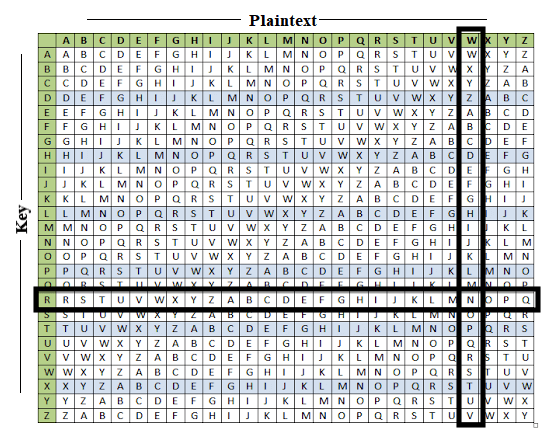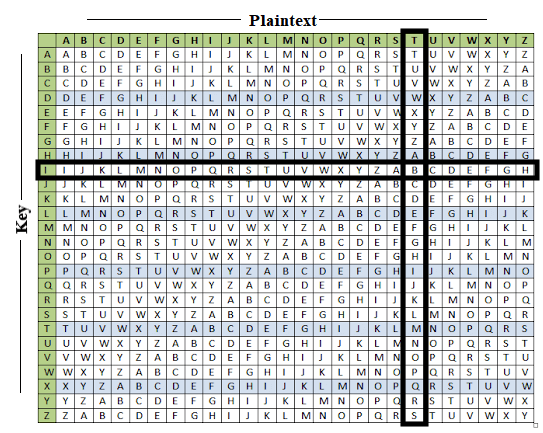# The Vigenere Cipher

## Introduction

The Vigenere cipher is a type of polyalphabetic cipher. Check out the Random Cipher, the Shift Cipher, or the Keyword Cipher for examples of a monoalphabetic cipher. A monoalphabetic cipher is a substitution cipher where the letters in the plaintext will always encrypt to the same letter. An example would be if "A" always encrypts to "H", "B" always encrypts to "Z", etc. A polyalphabetic cipher is one where you use different substitutions throughout the encryption process. For example, it's very possible that "A" encrypts to "H" and the next "A" encrypts to "T". The Vigenere is probably the most famous one; however, the Engima Machine from World War II is another quite famous type of polyalphabetic cipher.

It's important at this stage that you understand the Shift Cipher in order to understand the Vigenere cipher. In fact, the Vigenere cipher is basically a shift cipher, except each letter will use a different shift key. For example, a shift cipher with a key shift of 4 means every letter in the plaintext is shifted to the right by 4. This means "A" -> "E", "B" -> "F", etc. And this never changes throughout the encryption process. The Vigenere cipher could have the first letter of the plaintext shift by 4, the second letter by 8, the third letter by 6, and so on.

## How it works

Firs thing you need to do is have a password. It can be a regularly used word, a name, place, food, or even a random string of letters. In general people will choose something they can remember. For our example, I will use the password "WIZARD".

### Review of Shift Cipher

As mentioned before, you will need to understand the Shift Cipher. Here's a quick recap of the shift cipher:

• The shift key in a Shift cipher can be a number 0...25 or a letter A...Z.
• If you were told the Shift Key = "V", you would convert that to its corresponding number 21.
• 0 = A, 1 = B, 2 = C, 3 = D, 4 = E, etc.
• If the shift key was 21 or "V", you're substitution tables would look like this:

### Substitution (Ciphertext)

#### Key Shift of 21 ("V")

• Here's how we would show the encryption process for the plaintext ATTACK AT DAWN.

 Plaintext: A T T A C K   A T   D A W N Shift Key: 21 ("V"): V V V V V V   V V   V V V V Ciphertext: V O O V X F   V O   Y V R I

## Setting up the Vigenere Cipher

As you saw above, we placed the shift key below the letters of the plaintext. It was a way to tell us how to shift the letters. In the shift cipher, the shift is always the same. With the Vigenere cipher, the shifts change with each letter. Here's how we set it up.

Recall our password is WIZARD. Instead of placing "V" below each letter of the plaintext, we place the letters from WIZARD. Continue writing WIZARD until you reach the end of the plaintext.

 Plaintext: A T T A C K   A T   D A W N Password: WIZARD W I Z A R D   W I   Z A R D

## Perform the shifts

• The first letter in the plaintext "A" will use a shift of "W" (22). So "A" -> "W".
• The second letter in the plaintext "T" will use a shift of "I" (8). So "T" -> "B".
• The third letter in the plaintext "T" will use a shift of "Z" (25). So "T" -> "S".
• And so on...

Notice how the "T"s in ATTACK are encrypted to different letters!

 Plaintext: A T T A C K   A T   D A W N Shift Key: 21 ("V"): W I Z A R D   W I   Z A R D Ciphertext: W B S A T N   W B   C A N Q

## Is there a quick way to determine each shift?

There certainly is. When I encrypted the above message, I had to keep changing the shift key on my Shift cipher tool to match the corresponding letter in WIZARD. Instead of that, we have something called a Vigenere Cipher Table. It allows us to look up the plaintext letter, the Shift, and the corresponding ciphertext letter.Let's try it with the "W" in ATTACK AT DAWN. "W" has a shift key of "R". Find the "W" in the plaintext row, find the "R" in the key column, and locate their intersection.## How do we decrypt?

Set up the a table with the ciphertext and password.

 Ciphertext: W B S A T N   W B   C A N Q Shift Key: 21 ("V"): W I Z A R D   W I   Z A R D

Let's start with the first few letters of the ciphertext "W B S"">

• First, find the KEY "W" in the key column. Scroll across until you find the ciphertext letter "W". Scan to the top of that column to get the corresponding plaintext letter "A".• Let's do the next letter in the ciphertext "B". It's corresponding key is "I". Find the KEY "I" in the key column. Scroll across until you find the ciphertext letter "B". Scan to the top of that column to get the corresponding plaintext letter "T".
•• Let's do the next letter in the ciphertext "S". It's corresponding key is "Z". Find the KEY "Z" in the key column. Scroll across until you find the ciphertext letter "S". Scan to the top of that column to get the corresponding plaintext letter "T".
•Continue doing this until you've decrypted the entire ciphertext.

 Ciphertext: W B S A T N   W B   C A N Q Shift Key: 21 ("V"): W I Z A R D   W I   Z A R D Plaintext: A T T A C K   A T   D A W N